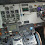# How to Calculate SMPS Transformer - Formula

Some people may still be confused about how the rules for the SMPS transformer or commonly called ferrite transformers.

Previously there were several rules for selecting wire diameters based on the frequencies used.
After knowing the wire that is used based on the frequency of the MOSFET driver that is used now we see first the formula determines the primary winding (Np).
Description
Npri = primary winding
Vin = Effective Input Voltage
f = frequency
Bmax = maximum flux density 1200 - 2000
Ac = Effective Cross Sectional Area (see datasheet of each type of transformer core (example EI33 = 1.19, ETD39 = 1.25).

For example, the above formula is specifically for push-pull topology.

For example, I want to make an inverter transformer with specifications:
Effective Vinput = 12v
Minimum Vinput = 10,5v
Max output = 330v
Normal Vout = 220v

Driver frequency = 50KHz or 50000Hz
then the primary (Np) and secondary (Ns) are?
I previously set the Bmax I want to use is 1600 (range Bmax 1200-2000) and uses EI33 core type with Ac 1.19

Np = (12v x100000000) / (4x50000x1600x1,19)
Np = 3.15 my turn rounds to 3 turns

check the Bmax value again not to be less than or more than the adjusted range above. then if I turn rounds into 3 (Np = 3).

Bmax = (12v x100000000) / (4x50000x3x1,19)
Bmax = 1680 (still in the range 1200-2000)

Now we calculate Primary Turn (Ns). because the output voltage can be adjusted or stabilized by setting the percentage duty cycle, if there is a voltage input drop to a minimum of 10.5v, the duty will increase to a maximum of 98% to maintain normal output stability at 220v or at least above 200v. For that N = 330v / (10,5v x 98% duty) = 32 so
Ns = N x Np
Ns = 32 x 3
Ns = 96 turns

To optimize the voltage-current, you can double the number of wires in accordance with the power and capacity of the bobbin.
Suppose you make a transformer with an output power of 300 watts, then 300watt / 220v = 1.4Amper and see the wire that can be used with a 50KHz frequency in the AWG table above. for example the wire used is 0.5mm then 1.4 Amp / 0.5 = 2.8 or rounded down or upward to be 2 or 3 double wire and of course the coil must be 2 or 3 times more primary wire used than secondary because Vin 12v then 300w / 12v = 25A so that the primary transformer wire does not overheat it must be joined into several wires in accordance with the bobbin ability or the capacity of the transformer bobbin.

## 6 comments for "How to Calculate SMPS Transformer - Formula"

1.Wow, the article is really good. I learned a lot about how to Calculate SMPS Transformer - Formula

2.good, very good

3.Thanks for the info, very informative in DIY of SMPS.

4.Thanks . Good article

5.Muy buena la explicación del tema para calcular estos transformadores

6.Is this formula work for flyback topology